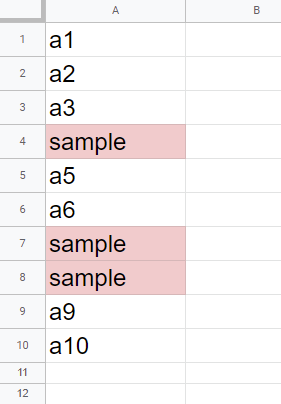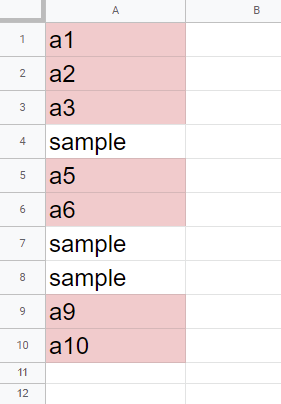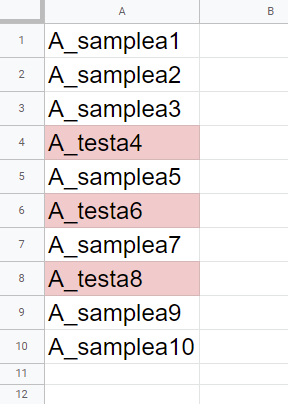Gists

There is Class TextFinder in Spreadsheet service for Google Apps Script. Ref The TextFinder can search and replace the texts in the Spreadsheet using Google Apps Script. There is the method for createTextFinder in Class Spreadsheet, Class Sheet and Class Range. When these methods are used, you can search and replace the texts for all sheets in a Spreadsheet, the specific sheet, and the specific range in the specific sheet.

In this post, I would like to introduce the sample scripts for taking advantage of TextFinder.

I think that TextFinder is the strong method for searching values in Google Spreadsheet. Ref So I think that when this can be taken advantage of, the process cost of the script will become low.

## Sample script 1

This sample search searchText from all cells of all sheets in a Google Spreadsheet. And the result value is returned as the range list including A1Notation.

const searchText = "sample";
.createTextFinder(searchText)
.matchEntireCell(true)
.findAll()
.map((r) => '${r.getSheet().getSheetName()}'!${r.getA1Notation()});
console.log(rangeList);

• When replaceAllWith("updatedText") is used instead of findAll(), all searched cells are replaced with updatedText.

## Sample script 2

This sample search searchText from all cells of the specific sheet in a Google Spreadsheet. And the result value is returned as the range list including A1Notation.

const searchText = "sample";
const sheetName = "Sheet1";
.getSheetByName(sheetName)
.createTextFinder(searchText)
.matchEntireCell(true)
.findAll()
.map((r) => r.getA1Notation());
console.log(rangeList);

• When replaceAllWith("updatedText") is used instead of findAll(), all searched cells are replaced with updatedText.

## Sample script 3

This sample search searchText from the specific range on the specific sheet in a Google Spreadsheet. And the result value is returned as the range list including A1Notation.

const searchText = "sample";
const sheetName = "Sheet1";
const range = "A1:A10";
.getSheetByName(sheetName)
.getRange(range)
.createTextFinder(searchText)
.matchEntireCell(true)
.findAll()
.map((r) => r.getA1Notation());
console.log(rangeList);When this sample sheet is used, ["A4","A7","A8"] is retrieved.

## Sample script 4

TextFinder can also search the texts using the regex. This sample search regex from the specific sheet in a Google Spreadsheet. In this case, the cells which don’t have the text of sample in the top letter are retrieved. And the result value is returned as the range list including A1Notation. This result is the opposite result from “Sample script 3”.

const regex = "^(?!sample).+$"; const sheetName = "Sheet1"; const range = "A1:A10"; const rangeList = SpreadsheetApp.getActiveSpreadsheet() .getSheetByName(sheetName) .getRange(range) .createTextFinder(regex) .useRegularExpression(true) .matchEntireCell(true) .findAll() .map((r) => r.getA1Notation()); console.log(rangeList);When this sample sheet is used, ["A1","A2","A3","A5","A6","A9","A10"] is retrieved. ## Sample script 5 This sample search searchText from all cells of all sheets in a Google Spreadsheet. And when searchText is found, true is returned. When that is not found, false is returned. const searchText = "sample"; const search = SpreadsheetApp.getActiveSpreadsheet() .createTextFinder(searchText) .matchEntireCell(true) .findNext() ? true : false; console.log(search);  • This situation can be also achieved for the specific sheet and the specific range. ## Sample script 6 This sample script searches the 1st empty cell of the specific column of the active sheet. When ^$ is used as the regex for TextFinder, the empty cells can be retrieved. This sample script retrieves the 1st empty cell of the specific column.

const columnNumber = 2; // 2 is column "B".
.createTextFinder("^$") .useRegularExpression(true) .matchEntireCell(true) .findNext(); const rowNumber = search ? search.getRow() : sheet.getLastRow() + 1; console.log(rowNumber);  ## Sample script 7 This sample script searches the cells including test from “A1:A10” of the active sheet, and the values of searched cells are retrieved. const searchText = "test"; const sheetName = "Sheet1"; const range = "A1:A10"; const values = SpreadsheetApp.getActiveSpreadsheet() .getSheetByName(sheetName) .getRange(range) .createTextFinder(searchText) .useRegularExpression(true) .findAll() .map((r) => r.getValue()); console.log(values);  When this script is used for the large range and the many sheets, the process cost will become high. In that case, you can reduce the process cost by using Sheets API as follows. When you use the following sample script, please enable Sheets API at Advanced Google services. Ref When Sheets API is used, the values can be retrieved from the individual cell ranges by one API call. const searchText = "test"; const sheetName = "Sheet1"; const range = "A1:A10"; const ss = SpreadsheetApp.getActiveSpreadsheet(); const ranges = ss .getSheetByName(sheetName) .getRange(range) .createTextFinder(searchText) .useRegularExpression(true) .findAll() .map((r) => '${sheetName}'!${r.getA1Notation()}); const values = Sheets.Spreadsheets.Values.batchGet(ss.getId(), { ranges, }).valueRanges.flatMap(({ values }) => values.flat()); console.log(values);When this sample sheet is used, for both scripts, the values of [ 'A_testa4', 'A_testa6', 'A_testa8' ] are retrieved. ## Sample script 8 The sample input and output situations are as follows. ### Input situation This value is put to the cell “A1” of “Sheet1”. sample=sample1,sample=sample2,sample=sample3  ### Output situation The above value of the cell “A1” are changed to the following situation. (sample1,sample2,sample3)  ### Sample script The sample script for achieving above situation is as follows. const sheetName = "Sheet1"; SpreadsheetApp.getActiveSpreadsheet() .getSheetByName(sheetName) .getRange("A1") .createTextFinder("sample\\=(.+),sample\\=(.+),sample\\=(.+)") .useRegularExpression(true) .replaceAllWith("($1,$2,$3)");